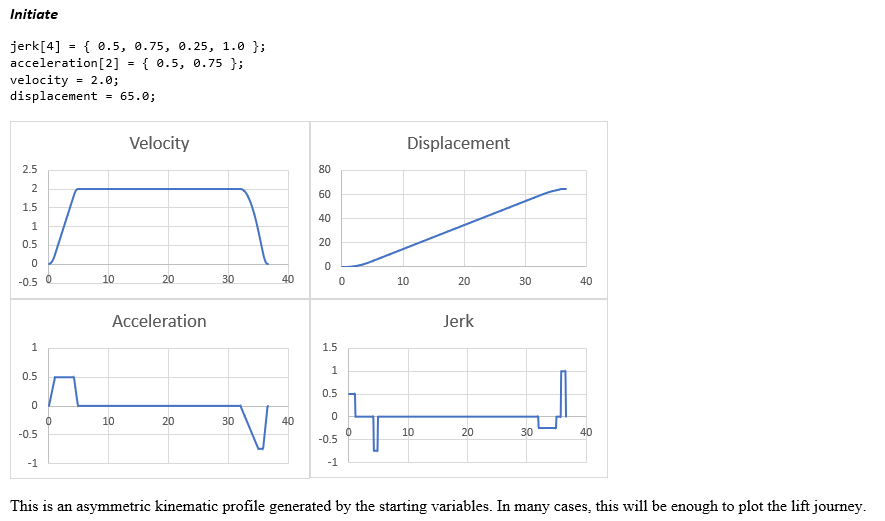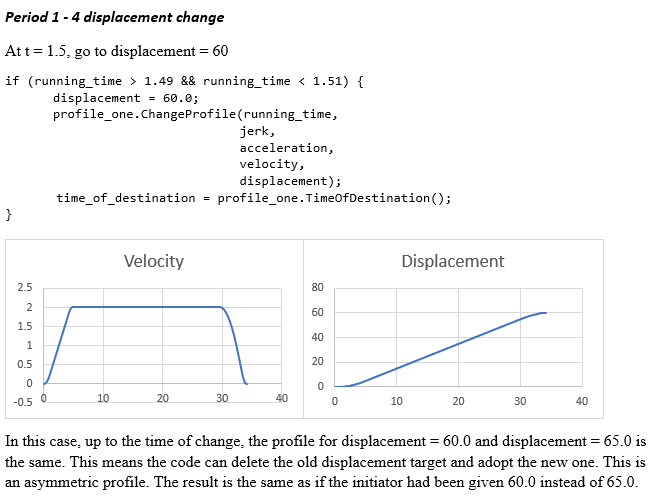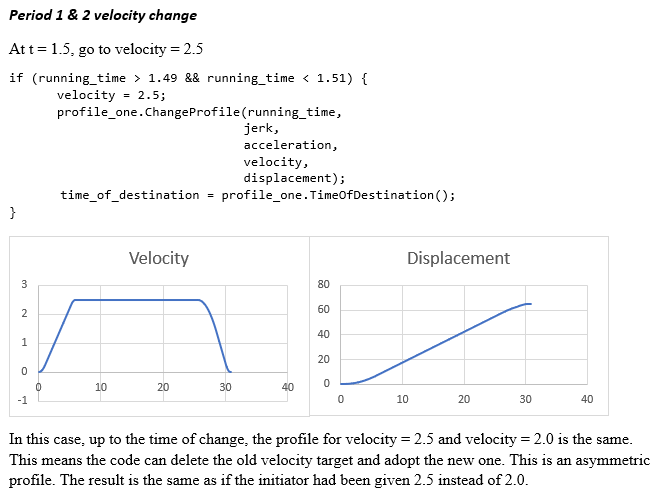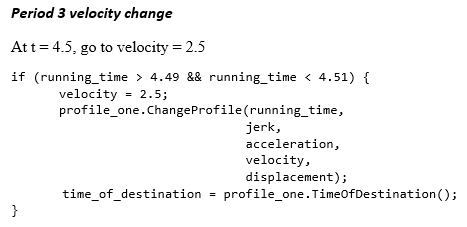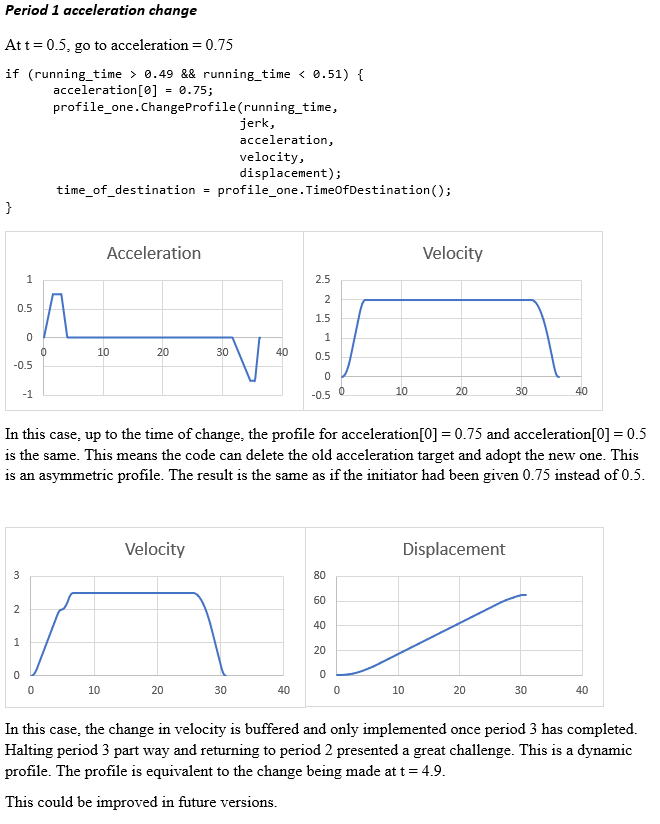## Dynamic extension for Ideal Kinematics

Matthew Appleby
Richard Peters

Peters Research Ltd, UK

Keywords: kinematics, lift, elevator, dynamic profile, ideal lift kinematics, twin lift system, 2 cars per shaft, multi, multi-dimensional lift system, simulation

Abstract. This paper presents a new set of equations for modelling kinematic profiles using a combination of mathematical and computational techniques. The implementation of these equations will extend the capabilities of the kinematic model to produce asymmetric and dynamic profiles. This will provide a more accurate model for standard lift systems as well as enable the modelling of more complex systems.

# 1           Introduction

Lift kinematics is the study of a lift car in a shaft without reference to mass or force. Variable speed drives can be programmed to match reference velocity profiles. This paper will show a set of equations which can be used to model a lift and produce a kinematic profile which can be used as a reference for lift simulations and physical lifts.

In previous papers regarding lift kinematics, equations have been produced which model symmetric profiles  and asymmetric profiles . This paper will show a new set of equations which will model dynamic profiles.

## 1.1Symbols

 d Lift journey distance (m) vphase Velocity at end of given phase (m/s) final_phase Final phase in car journey aphase Maximum acceleration (m/s2) jphase,0 Maximum jerk0 in given phase (rate ofchange of acceleration) (m/s3) jphase,1 Maximum jerk1 in given phase (rate ofchange of acceleration) (m/s3) pphase,0 Phase start time/period 0 start time pphase,1 Period 1 start time pphase,2 Period 2 start time pphase,3 Period 3 start time D(t) Distance travelled at time t (m) V(t) Velocity at time t (m/s) A(t) Acceleration at time t (m/s2) J(t) Jerk at time t (m/s3) dmin Minimum displacement

## 1.2             Definitions

symmetric profile
the profile of a journey with one target velocity, the same acceleration as
deceleration and four identical jerk values. See Fig. 1

asymmetric profile
the profile of a journey with one target velocity but different target
acceleration and deceleration or differing jerk values. See Fig. 2

dynamic profile
the profile of a journey with multiple target velocity values. Acceleration
and jerk can also vary. See Fig. 3

period
a section of time where the lift is at constant jerk. See Fig. 4
& Fig. 5

phase
a section of time where the lift is changing from one speed to another
including the time it remains at its final speed. A period starts and finishes
with acceleration of 0 and contains a maximum of four periods. See Fig. 4
& Fig. 6

journey
a section of time where the lift is changing displacement from when the
lift begins to move, to when it reaches its destination. Contains a minimum of
two phases. See Fig. 4

## 1.1Three types of profile

There are three types of
kinematic profile that will be referred to in this paper:

### 1.1.1Symmetric Profile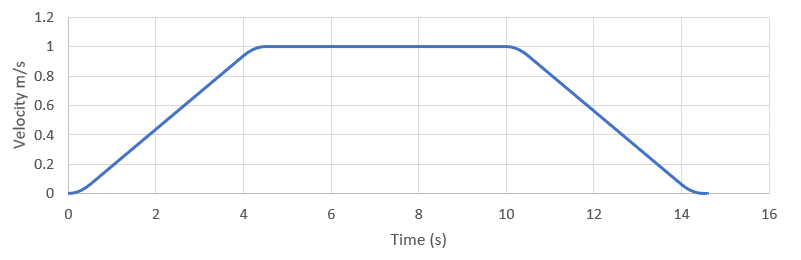Fig. 1 Symmetric Profile

Fig. 1 shows a profile produced when a symmetric model has been used to plot the
kinematic profile of a lift. This assumes that there will only be one target
velocity and thus the lift is unable to reduce or increase velocity even as the
surrounding environment changes. It also makes some assumptions about the
kinematic parameters:

 abs(j0,0) = abs(j0,1) = abs(j1,0) = abs(j1,1) (1) abs(a0) = abs(a1) (2) v0,0 = v1,1 = vfinal_phase,1  = 0 (3)
This is the most used profile model and the equations for it
can be found in ‘Ideal Lift Kinematics’ by Peters
 as well as CIBSE
Guide D Annex 2
. Most lift systems
aim for a symmetric profile to produce a smooth ride quality.

### 1.1.1Asymmetric Profile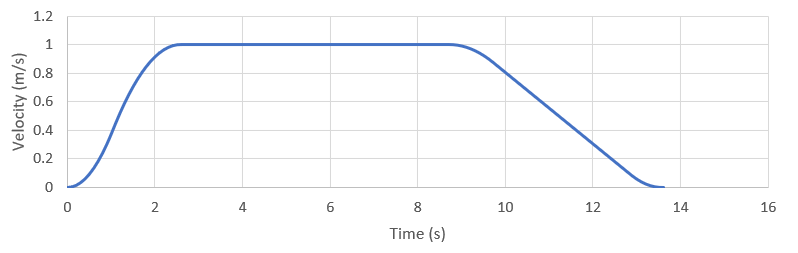Fig. 2 Asymmetric Profile

Fig. 2 shows a profile when an asymmetric model has been used to plot the kinematic
profile of a lift. Like the symmetric profile, this model assumes that there
will only be one target velocity, however, it allows for different acceleration
and deceleration values as well as differing jerk values.

These profiles can be produced using the equations given in
‘Quality and quantity of service in lift groups’ by Gerstenmeyer . The equations are
an extension to the previous ideal lift kinematic equations meaning they can
model symmetric and asymmetric equations.

Applications include the modelling of lift cars which have a
different acceleration to deceleration, often due to poor motor control. Asymmetric
profiles can also be applied to improve lift system performance where there are
two cars per shaft as cars sharing a shaft impose additional safety constraints.
For example, a lower deceleration may be used when the lower and upper cars
need to be moved closer than allowed by the preferred safety distance.

### 1.1.1Dynamic Profile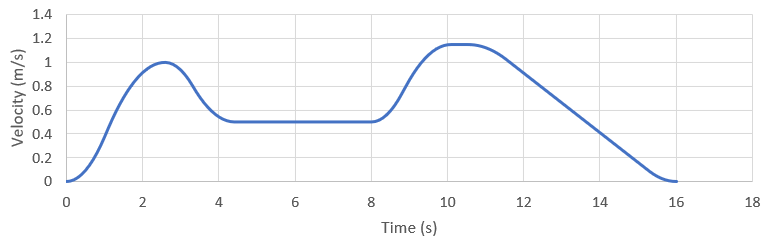Fig. 3 Dynamic Profile

Fig. 3 shows a profile when a dynamic model has been used to plot the kinematic
profile of the lift. This level of complexity is achieved when the lift changes
its target velocity mid journey to slow down or speed up the car based on the
location of the other cars in the shaft. Each update contains a target
velocity, jerk0, jerk1, acceleration and a displacement. These update
parameters either replace current/future parameters or create a new phase.

This model provides more flexibility than the asymmetric
profile, giving more control of the location and motion of the lift car to
improve the performance of the system. For example, if a higher car traveling
up has been delayed longer than expected, a lower car might need to reduce its
velocity until the path is clear for it to accelerate back up to speed. This slow
down option makes it more acceptable to start the lower car before the upper
car is guaranteed to be moving out of the way. The increase in performance is
particularly valuable to multi-dimensional lift systems with more than two lift
cars per shaft. .

## 1.1Three segments of a profile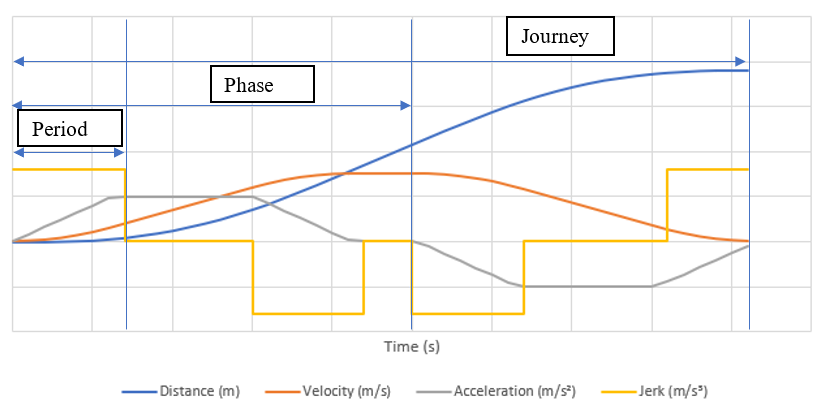Fig. 4 Period, Phase
and Profile labelled profile

Fig. 4 shows the kinematic profile of one symmetric journey. The first period and
phase, and the journey are labelled.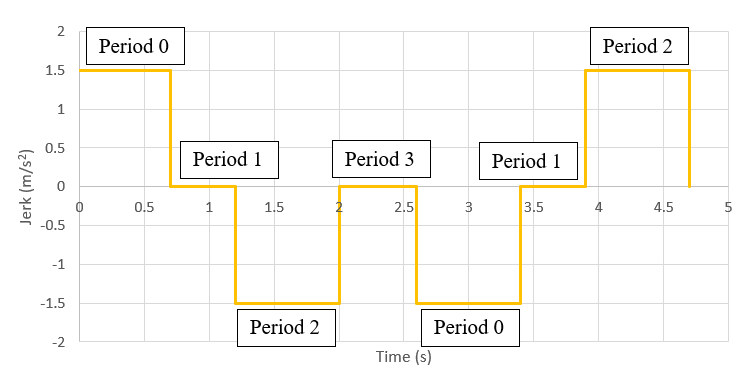Fig. 5 period labelled profile

Fig. 5 shows the jerk profile of one symmetric journey. Each period has a different jerk value and the period count resets to 0 when the car enters a new
phase.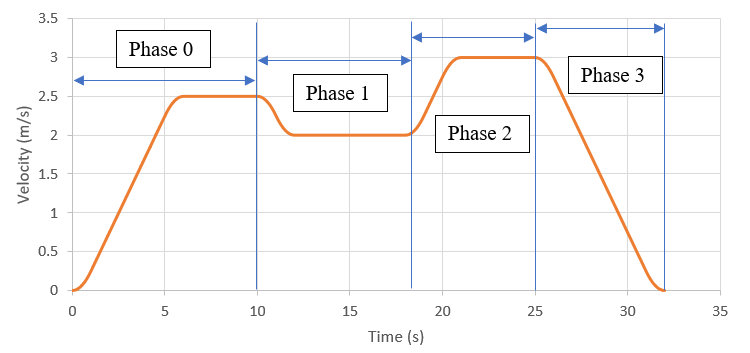Fig. 6 phase labelled profile

Fig. 6 shows the velocity profile of a dynamic profile. The phases have been labelled to demonstrate where phases start and stop on dynamic profiles.

# 1Overview of previous research

## 1.1Previous Work

### 1.1.1Analytical Method

Peters provided a set of equations which model the kinematic
profile of a symmetric journey . Each journey is
divided into seven periods, each with their own set of equations. Each equation
does the entire integration including the addition of the starting value at the
previous period. This model provides a straightforward set of individual
equations which do not approximate each integration. These equations are
transparent and functional but very long and lack flexibility. This is also the
method described in Annex 2 of Guide D .

Gerstenmeyer provided a set of equations which model the kinematic profile
of an asymmetric journey . This uses similar
logic to the symmetric model however allows four different jerk inputs and two
different acceleration values for the two phases involved. Whilst this improves
the flexibility of the model, it also makes the equations even longer and harder
to implement.

In the cases where a lift cannot reach the inputted velocity
or acceleration, Peters proposed alternative models called ‘case B’ and ‘case
C’ , Gerstenmeyer however proposed using the same
equations by first reducing the velocity and acceleration to the maximum
possible values that can be reached .

### 1.1.2Computational Method

Computational integration methods include quadrature rule,
generalised midpoint rule, adaptive algorithms and extrapolation. These methods
use calculations which approximate integration to find the profile values
without long equations. This method is far more flexible than the analytical
method as it does not rely on period separations. However, the approximation
required in the computational method decreases the accuracy of the profile. 

## 1.2Authors’ contribution

The authors have derived an alternative set of equations
which map onto the existing equations but allow for more flexible input
parameters thus allowing the controller to have more flexibility over the shape
of a lift’s kinematic profile. The new equations use a combination of analytical
and computational techniques and use the Gerstenmeyer method for dealing with
invalid input parameters .

# 2Functional Method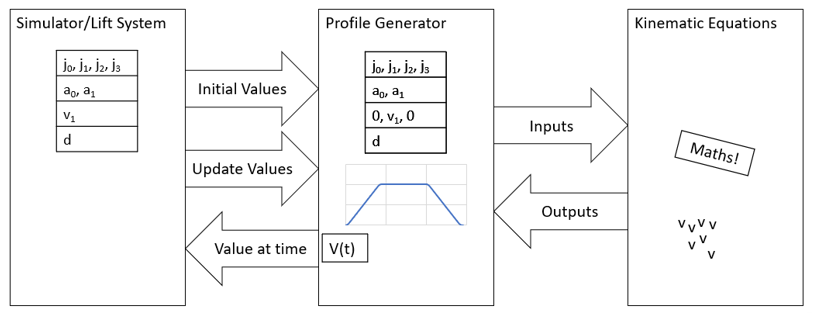Fig. 7 Logic diagram instantiation

The three boxes in Fig. 7 represent the three coded classes. The first box represents the Simulator or
Lift System and will be the calling code. The Profile Generator is a class
which takes some parameters upon instantiation of an object. The resulting
object contains a set of public arrays which plot the profile and can be used
by the calling code. The Profile Generator uses the static functions from the
Kinematic Equations class to produce the profile. This Kinematic Equations
class can be used by other coded classes to perform all the calculations our
previous symmetric Kinematic Equations class could do and more.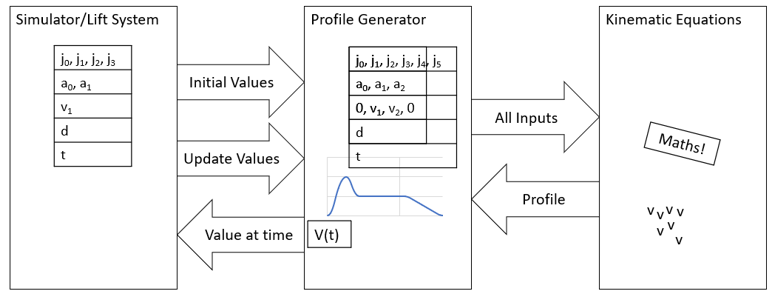Fig. 8
Logic diagram update

For dynamic profiles, the calling code can invoke an update
method on the profile object with a new set of inputs including the time at
which the update was sent. This will modify the input parameters and then
modify the profile arrays.

4           Mathematical Method

## 4.1              Phase start times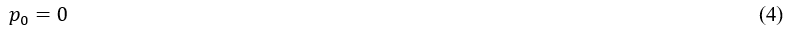p0 will only equal
0 in the first phase. For all the middle phases in a dynamic profile, p0
will be determined by the time at which the update is sent. For the final phase
p0 is calculated by finding the difference between the target
displacement and the minimum displacement and then calculating how long the
lift must travel at its final velocity (vfinal_phase) to reach the
target displacement.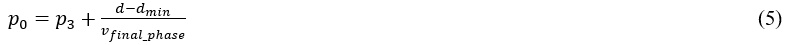The period start times p1-3 are calculated using the
following equations which can be derived from equations (8-1/2/3) in .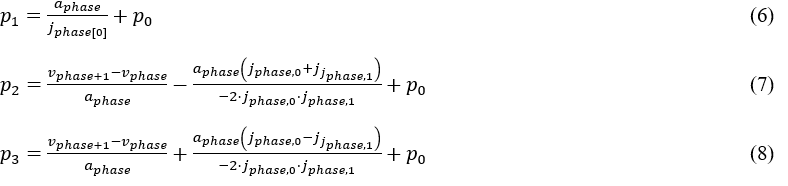## 4.2Kinematic equations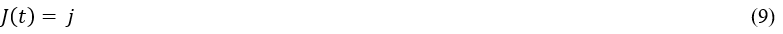Starting with a constant jerk, acceleration can be
found by integrating the jerk value with respect to time and adding the
starting acceleration at the beginning of the current period (pthis)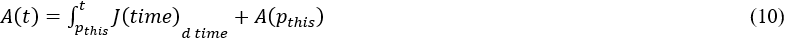Velocity can be calculated two ways, by either adding the
velocity change onto the starting velocity (V at pthis), or by
removing the velocity change from the target velocity (V at pnext).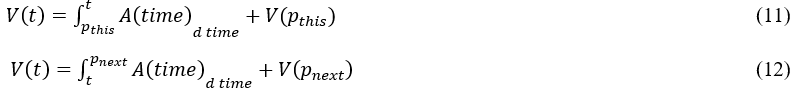Displacement is the integration of velocity plus the
displacement at the beginning of the current period.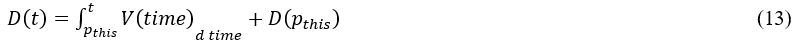See Appendix A
for the full equations for each period.

##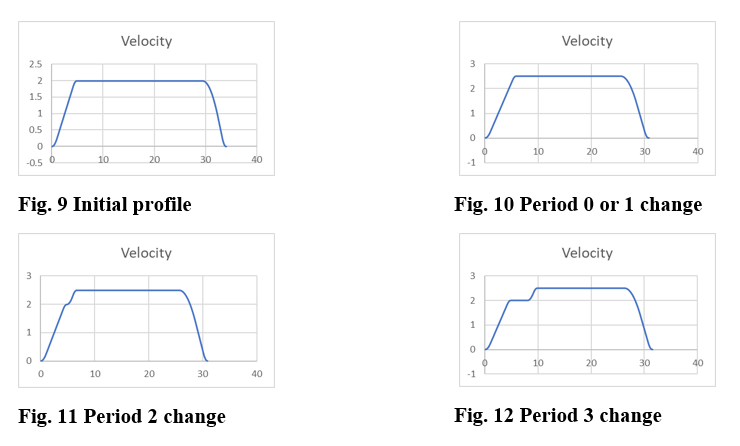If the car is in period 0 or 1, a command to increase the
velocity can be accepted. The lift will continue accelerating to the higher
speed. For example, in
Fig. 9
the initial profile was set to reach a velocity of 2m/s. Because the target velocity
was changed to 2.5m/s before the end of period 1, the new speed profile is the
same as if the higher speed had been commanded at the beginning of the trip,
see
Fig. 10.

If, however, the lift has entered period 2 and the same
command is sent to increase the velocity to 2.5m/s, the lift must finish its
current phase before starting to accelerate again up to a higher velocity thus
creating a new phase, see Fig. 11.
The reason for completing the period is to avoid the added complexities required
in changing the jerk mid-period whilst acceleration is not constant.

If the update is sent when the lift is no longer
accelerating but is moving at a constant speed in period 3, a new phase is
created. The new phase starts at the time the update was sent as seen in Fig. 12.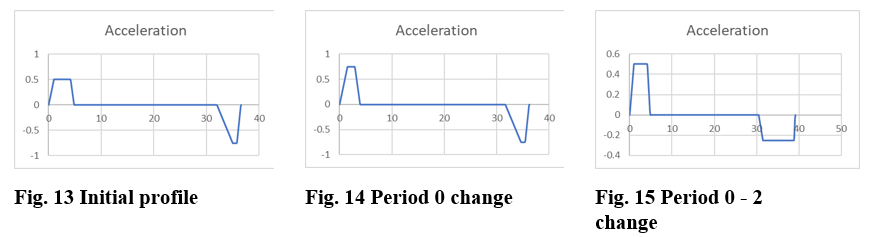If a valid update is sent in period 0 to increase
acceleration, the car will continue to jerk up to a higher acceleration. For
example, in Fig. 13
the initial profile was set to reach an acceleration of 0.5m/s2.
Because the acceleration was changed to 0.75m/s2 before the end of
period 0, the new acceleration profile will be the same as if the higher
acceleration has been commanded at the beginning of the trip, see Fig. 14.

If a valid update is sent to change the deceleration at any
time before the final phase, the working parameters will be updated, see Fig. 15.
This update must arrive before the car enters period 3 and, if the deceleration
is decreased, before the car would have needed to enter period 3 with the new
deceleration.

If an update is sent to increase the displacement and the
lift has not entered its final phase, this will always be valid, and the
profile should be adjusted accordingly. If the update requests a decreased displacement,
it must first be calculated if the lift can stop before the requested
displacement in the given jerk, acceleration and velocity.

### 4.4.1High acceleration

When the car cannot reach the input acceleration and then
acceleration is too high and must be reduced. This is done using equation 8-12 .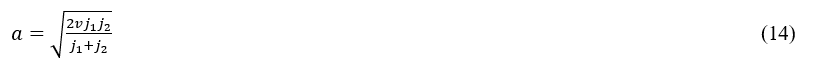### 4.4.2High velocity

When the car cannot reach the input velocity and then slow
down again without surpassing the destination displacement, the velocity is too
high and must be reduced.

Due to the use of computational techniques in this method, an
analytical equation has not been produced to solve for the maximum possible
velocity. Instead, a computational method is used which implements trial and
improvement to find the new velocity value.

### 4.4.3Low displacement

When the car is mid journey and is sent an update to stop at
a displacement lower than the minimum stopping distance, this kinematic update
must be rejected.

### 4.4.4Late update

When the car is mid journey and is sent an update to change
a parameter that has already been used or is impossible to implement, this
kinematic update must be rejected. One example of this kind of update is when a
command is sent in the final phase of a lift’s journey.

# 5Application

## 5.1More accurate lift traffic analysis

When modelling lift kinematics, it is currently assumed that
the acceleration is the same as the deceleration and that all four jerk values
are the same. In a real lift system, acceleration and deceleration can vary in
a single journey as seen by accelerometer data. As more data is analysed, more
accurate models can be produced when calculating lift system performance.

## 5.2Lift systems with two cars per shaft

When two cars share a shaft, the system performance can be
improved by giving each car the option to follow a deliberate asymmetric
profile thus improving the performance of the system .

## 5.3Multi-dimensional lift system

For multi-dimensional systems, cars can be given the option to
change velocity based on the location of other cars in the system. This can
prevent unnecessary stops, improving user experience, and can reduce waiting
times, improving performance.

## 5.4Improvements to monitoring

Lift performance measurement tools  currently try to map
the lift’s motion to a symmetric profile. Not only will this new model allow
monitoring of deliberately asymmetric lifts, data from which will improve
simulation inputs, it will also allow for the monitoring of poorly adjusted
symmetric lifts thus enhancing maintenance.

# 6Conclusion

This paper provides a set of equations which accurately
model a lift’s kinematic profile for symmetric, asymmetric and dynamic
journeys. This will enable simulations to model lift motion more accurately as
well as improve the performance of lift dispatchers. These improvements will be
most noticeable in lift systems with two lift cars per shaft and with multi-dimensional
lifts. The equations will also enable improvements in surveying, maintenance
and many more technologies.

Currently the solution uses a computational brute force
technique to find the maximum velocity and quickest stop floor at any given
time slice. There are improvements which can be made to these solutions which
will save time and processing power. Although time is less of a concern when
simulating a lift system, the need for efficient algorithms becomes more
crucial for dispatching and monitoring technologies.

# 7ACKNOWLEDGEMENTS

The authors acknowledge the work of Gabrielle Anderson and
her research into the field of lift kinematics with multiple maximum velocities
and with separate acceleration and deceleration. The authors would like to
thank Nishad Deokar for checking the maths presented in this paper and continuing
the research surrounding dynamic kinematics.

# 8BIOGRAPHY

Matthew Appleby is a Software Engineer with Peters Research
Ltd. He is part of the team working on enhancements to Elevate, elevator
traffic analysis and simulation software, and related software projects. He
joined Peters Research in 2019 and is studying part-time for a Digital Degree
Apprenticeship.

Richard Peters has a degree in Electrical Engineering and a
Doctorate for research in Vertical Transportation. He is a director of Peters
Research Ltd and a Visiting Professor at the University of Northampton. He has
been awarded Fellowship of the Institution of Engineering and Technology, and
of the Chartered Institution of Building Services Engineers. Dr Peters is the
principal author of Elevate, elevator traffic analysis and simulation software.

# 9            References

  R. Peters, “IdealLift Kinematics,” in Proceedings of ELEVCON ’95, Hong Kong, 1995.  S. Gerstenmeyer,Quality and quantity of service in lift groups, University of Northampton,2018.  CIBSE, CIBSE GuideD: Transportation systems in buildings, 2020.  T. Croft,Mathematics for engineers, Pearson Prentice Hall, 2015.  R. Peters, “LiftPerformance Time,” in Proceedings of the 2nd Symposium on Lift andEscalator Technologies, Northampton, 2012.

# 10Appendix

## 10.1             Appendix A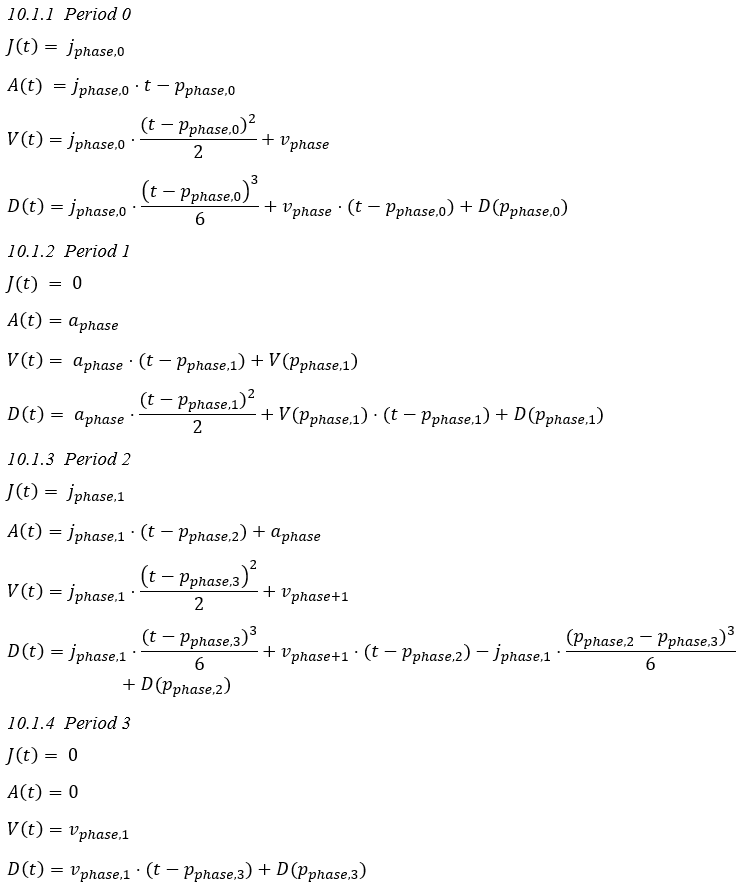## 10.2Appendix B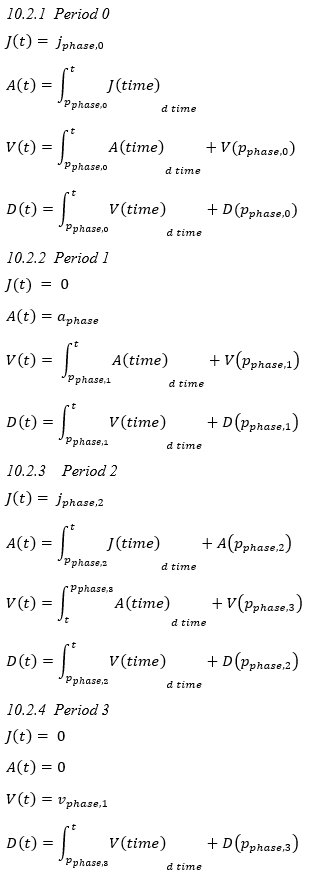## 10.3Appendix C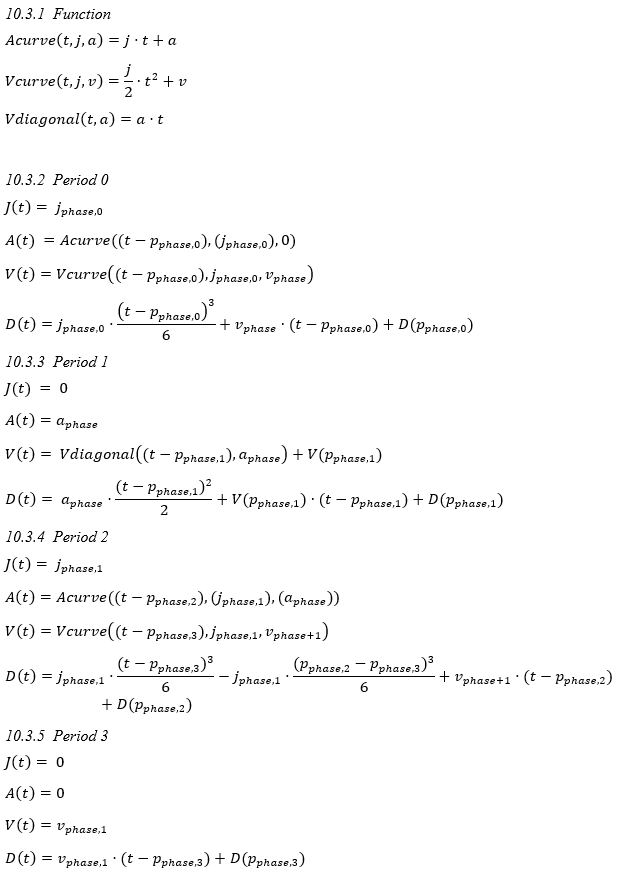## 10.4Appendix D

The following appendix contains examples of input parameters
and resulting profiles. They attempt to demonstrate all categories of profile
and can be used as test cases for any future developments.